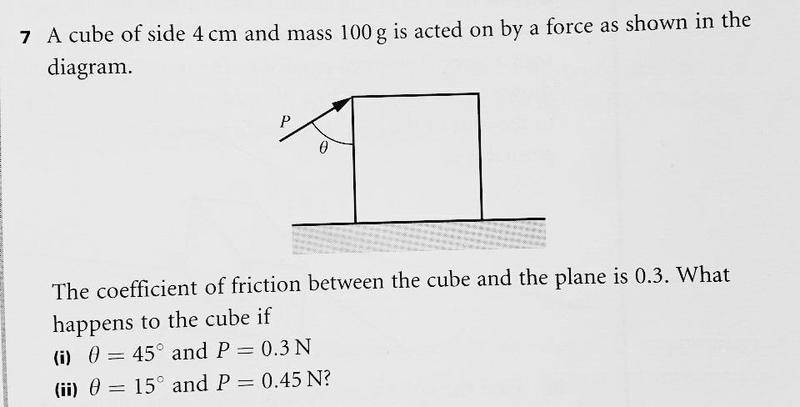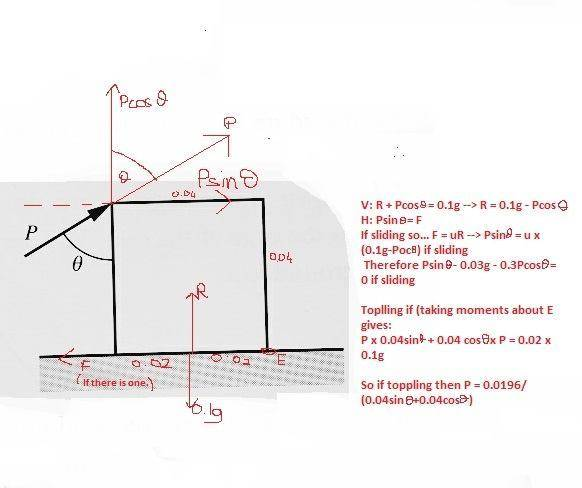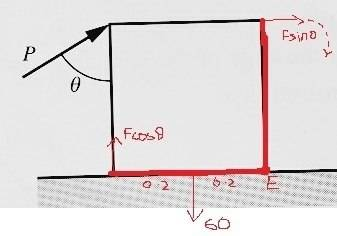# Does this cube slide or topple?

I have attached a copy of the full question and my diagram which I thought was correct but it does not give me the correct answer. The answer for (i) is that it stays put and for (ii) that it topples.

I do not get = 0 for any of them. I get some values... (i) -0.15 for slide, 0.346 for toppleLooking at your equation for moments about E, it looks as though you haven't correctly calculated the distance to to point of action of P. If the sides are of length 0.04m then what is the length of the diagonal from E to P?

Looking at your equation for moments about E, it looks as though you haven't correctly calculated the distance to to point of action of P. If the sides are of length 0.04m then what is the length of the diagonal from E to P?
Square root of 2 divided by 25?

So now I changed the equation to P = 0.0196/(SQrt(2)/25sinAngle + 0.04cosAngle) but it gives me answer of P = 0.287 for angel= 45 (i) , and 0.36788... for (ii) angle = 15, not the required values of P.

You need to use Pythagoras, the two sides of the cube (vertical and horizontal) give you two sides of a right angle triangle. There is an easier way to solve this problem though:

Often with these problems the easiest way to solve it is to take components of the two forces such that they are acting parallel. In this case weight acts vertically downward. So what component of P will give you a line of action parallel to that of the weight? Then simply solve by using the perpendicular distances.

(Also, bear in mind that you are not looking for an answer = 0, the method you are using is to set the moments equal to each other and find the value of P for which they are equal. This will give you the minimum value of P that will result in a toppling motion. If P is greater than this then the cube will topple, if it is less than the value found then it will not topple.)

You need to use Pythagoras, the two sides of the cube (vertical and horizontal) give you two sides of a right angle triangle. There is an easier way to solve this problem though:

Often with these problems the easiest way to solve it is to take components of the two forces such that they are acting parallel. In this case weight acts vertically downward. So what component of P will give you a line of action parallel to that of the weight? Then simply solve by using the perpendicular distances.

(Also, bear in mind that you are not looking for an answer = 0, the method you are using is to set the moments equal to each other and find the value of P for which they are equal. This will give you the minimum value of P that will result in a toppling motion. If P is greater than this then the cube will topple, if it is less than the value found then it will not topple.)

I have used phytagoras. the length we looking for is let us say c, c = Sqrt of (0.042 + 0.042)

My calculator said this was Sqrt of 2 over 25.

I have used phytagoras. the length we looking for is let us say c, c = Sqrt of (0.042 + 0.042)

My calculator said this was Sqrt of 2 over 25.

Sorry, I posted my last reply before I'd seen yours.

Okay, ignore my second solution. Now you've got the correct distance but you are adding the components of P in vertical and horizontal directions (cosθ and sinθ) rather than finding the component of P which acts perpendicular to the line connecting P and E. (which is the definition of a moment)

Sorry, I posted my last reply before I'd seen yours.

Okay, ignore my second solution. Now you've got the correct distance but you are adding the components of P in vertical and horizontal directions (cosθ and sinθ) rather than finding the component of P which acts perpendicular to the line connecting P and E. (which is the definition of a moment)

Okay I see what you mean but that gives me moments about E like these:
Let srt2/25 be c
of P x c = 0.1g x 0.02 --> P = 0.0196/c so.... the value of P in this case would be completely independent of angle..?

How am I meant to check if the angle when P = 0.3 is 45 if the value of P is constant...?

By constant do you mean "if it stays the same as the block tips"?

By constant do you mean "if it stays the same as the block tips"?
Yeah well it doesn't make sense to me because the bigger force there is, the more likely the block is to slide or topple so... how do I get my actual equation of P and angle after I know the moments? I don't get it.Here I have done the moments on this diagram. This is why I don't get why I need to do P forces. As you can see on this diagram, this is where I am coming from. The moments should be the equation I have given since Fcos and Fsin are negative forces, and 60N works against these forces. I have even used this method in a question before. I just don't understand why its not giving me the answer.

It's as if there was a certain inequality for angles when it topples and angle when it slides. But Idk how to work around it or figure out these values.

View attachment 100828 Here I have done the moments on this diagram. This is why I don't get why I need to do P forces. As you can see on this diagram, this is where I am coming from. The moments should be the equation I have given since Fcos and Fsin are negative forces, and 60N works against these forces. I have even used this method in a question before. I just don't understand why its not giving me the answer.

It's as if there was a certain inequality for angles when it topples and angle when it slides. But Idk how to work around it or figure out these values.

When resolving moments the forces all need to be taken along the same line from the pivot point, otherwise it's like trying to resolve around a corner. At the moment your 'Psinθ' is acting against friction whereas your 'Pcosθ' is the force acting against the moment of the weight. You only need the second one when resolving moments.

So you're saying I should be doing only Fcos a x 0.04 = 0.02 x 60?

When resolving moments the forces all need to be taken along the same line from the pivot point, otherwise it's like trying to resolve around a corner. At the moment your 'Psinθ' is acting against friction whereas your 'Pcosθ' is the force acting against the moment of the weight. You only need the second one when resolving moments.
So, would you say that component Fsin a is acting along the base, and so does not need resolving..?

So, would you say that component Fsin a is acting along the base, and so does not need resolving..?

Yes, exactly.

So you're saying I should be doing only Fcos a x 0.04 = 0.02 x 60?

I'm not sure where you got 60 from, the weight is 0.1*g, but yeah the LHS is correct if F is P and a is θ

Yes, exactly.

I'm not sure where you got 60 from, the weight is 0.1*g, but yeah the LHS is correct if F is P and a is θ
Ahh yeah sorry got mistaken with another question.

You should find for the first question that 0.3N is less than the threshold force that will produce toppling when the angle is 45 and that for part two 0.45N with an angle of 15 is greater than the threshold force